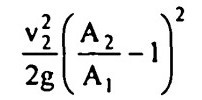Search

# Fluid Mechanics (81-100)

Updated: Apr 10, 2020

81. If x is the distance from leading edge, then the boundary layer thickness in laminar flow varies as

82. Stanton diagram is a log-log plot of friction factor against Reynolds number

83. The depth 4d below the free surface at which the point velocity is equal to the

average velocity of flow for a uniform laminar flow with a free surface, will be 0.577D

84. The boundary layer thickness in turbulent flow varies as

85. The distance y from pipe boundary, at which the point velocity is equal to

average velocity for turbulent flow, is 0.223 R

86. If a sphere of diameter 1 cm falls in castor oil of kinematic viscosity 10 stokes, with a terminal velocity of 1.5 cm/sec, the coefficient of drag on the sphere is

160

87. In case of an airfoil, the separation of flow occurs at the extreme rear of body

88. When an ideal fluid flows past a sphere total drag is zero

89. With the same cross-sectional area and immersed in same turbulent flow, the

largest total drag will be on a circular disc of plate held normal to flow

90. In which of the following the friction drag is generally larger than pressure drag? an airfoil

91. For hydro-dynamically smooth boundary, the friction coefficient for turbulent flow is dependent only on Reynolds number

92. The value of friction factor T for smooth pipes for Reynolds number 106 is

approximately equal to 0.01

93. For laminar flow in a pipe of circular cross-section, the Darcy's friction factor f

is inversely proportional to Reynolds number and indpendent of pipe wall roughness

94. Separation of flow occurs when the boundary layer comes to rest

95. The loss of energy due to sudden enlargement is given bywhere A1, V1 are area of cross-section and velocity at entry and A2, v2, are area of

cross-section and velocity at exit.

96. The ratio of average velocity to maximum velocity for steady laminar flow in circular pipes is 1/2

97. The distance from pipe boundary, at which ,the turbulent shear stress' is one

third the wall shear stress, is 2/3 R

98. The discharge of a liquid of kinematic viscosity 4 cm²/sec through a 8 cm dia

meter pipe is 3200π cm³/sec. The type of flow expected is laminar flow

99. The Prandtl mixing length is zero at the pipe wall

100. The velocity distribution for laminar flow through a circular tube varies parabolically with maximum at the centre